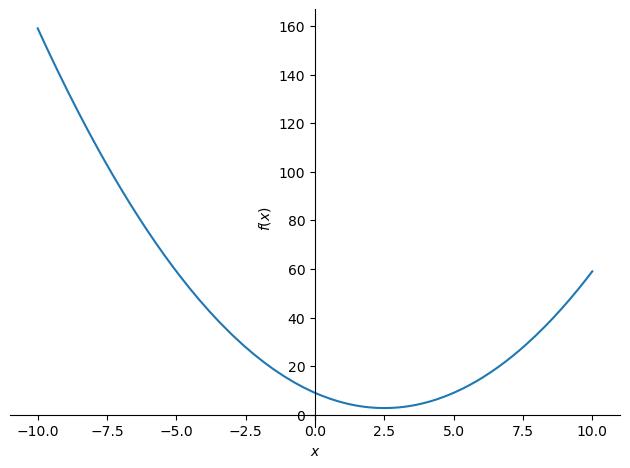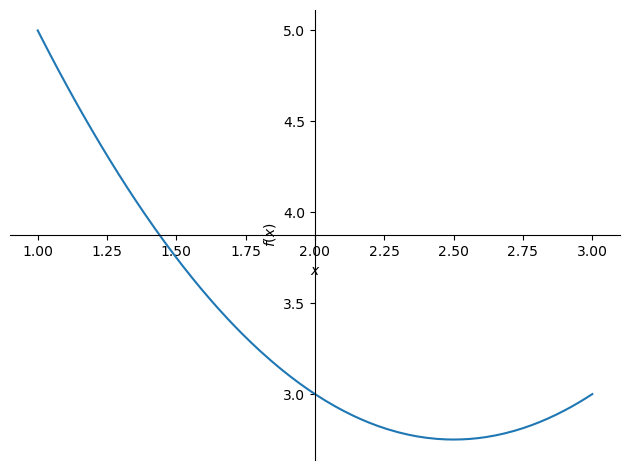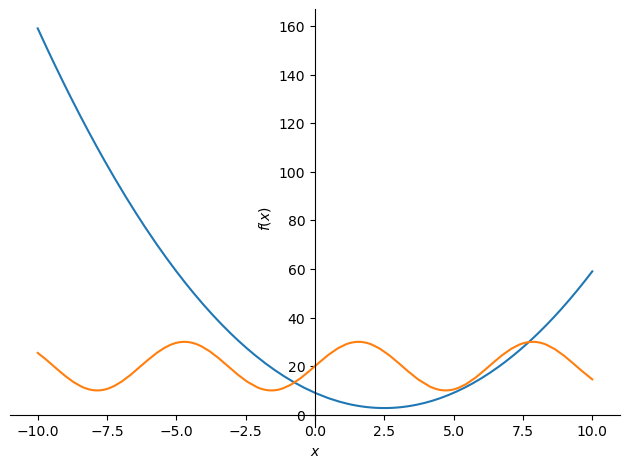# How to graph mathematical functions (in Python, using SymPy)

See all solutions.

Assume we have a mathematical formula and we would like to plot a graph of it using the standard Cartesian coordinate system.

## Solution

This answer assumes you have imported SymPy as follows.

1
2
from sympy import *                   # load all math functions
init_printing( use_latex='mathjax' )  # use pretty math output


You can write a formula and plot it in just a few lines of code.

1
2
3
var( 'x' )
formula = x**2 - 5*x + 9
plot( formula )1
<sympy.plotting.plot.Plot at 0x7f4b97b97520>


If you want to elimiate the extra bit of text after the graph, just assign the plot to a variable, as in p = plot( formula ).

By default, the graph always covers $x=-10$ to $x=10$. You can change those limits as follows.

1
plot( formula, (x,1,3) )   # just plot from x=1 to x=31
<sympy.plotting.plot.Plot at 0x7f4bbc097550>


You can also plot more than one function on the same graph.

1
2
formula2 = 10*sin(x) + 20
plot( formula, formula2 )1
<sympy.plotting.plot.Plot at 0x7f4b2dcd9c00>